# GMAT Math : Calculating ratio and proportion

## Example Questions

1 3 Next →

### Example Question #21 : Calculating Ratio And Proportion

An architect built a scale model of a new building she was designing. In the scale model, the base of the building is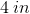wide by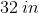long. If the actual building with have base length of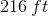, what will the width of the actual building be?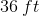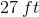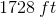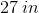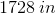Explanation:

We are asked to compare lengths of sides between a scale model and an actual building. This sounds like a ratio question. Set up our ratio so that our unknown is in the numerator. Make sure to set it up with similar sides together: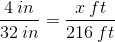Cross-multiply and simplify to find the correct answer: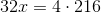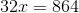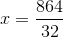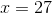So, the width of the actual building is.

### Example Question #22 : Calculating Ratio And Proportion

Leticia ran a half marathon (13.1 miles) inhours andminutes. Using her average running speed, how long would it take her to run a 10k (6.2 miles)?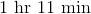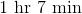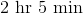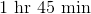Explanation:

We need to use her total time and distance to find her running pace. Once we do, we can solve the problem.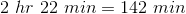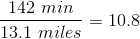That is, she ran at about a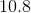-minute-mile pace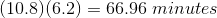So, it will take her about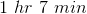### Example Question #23 : Calculating Ratio And Proportion

If a fabric costs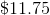a yard, how much willfeet cost?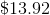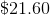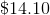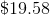Explanation:

We first need to determine how much one foot of the fabric cost. Remember, one yard is equivalent to three feet: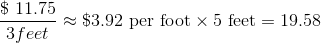So, five feet would cost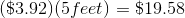1 3 Next →

Tired of practice problems?

Try live online GMAT prep today.